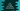# C++ program to merge two arrays into one array## C++ program to merge two arrays into one array:

In this post, we will learn how to merge two arrays into one single array in C++. We will write one program that will take the arrays as inputs from the user and merge them to one single array.

This program will take the inputs for the array one by one from the user.

### Algorithm to use:

We will have to create one new array and this array will hold the final merged array. It will follow the below steps:

• Take the size of the first array as input from the user.
• Take the size of the second array as input from the user.
• Create one new final array by adding the sizes of both of these arrays.
• Run one loop and read the contents of the first array from the user one by one. Run another oop and read the contents of the second array from the user similarly.
• Run two more loops and iterate over the contents of the first and second arrays. While iterating, insert the items to the final array. So, at the end of the program, the final array will hold the merged content of the two arrays.

### C++ program:

Let’s write down the program now:

``````#include <iostream>

using namespace std;

int main()
{
// 1
int firstArrSize, secondArrSize;

// 2
cout << "Enter the size of the first array: " << endl;
cin >> firstArrSize;

cout << "Enter the size of the second array: " << endl;
cin >> secondArrSize;

// 3
int firstArr[firstArrSize], secondArr[secondArrSize], finalArr[firstArrSize + secondArrSize];

// 4
cout << "Enter values for the first array: " << endl;
for (int i = 0; i < firstArrSize; i++)
{
cout << "Enter value for index " << i << " : " << endl;
cin >> firstArr[i];
}

cout << "Enter values for the second array: " << endl;
for (int i = 0; i < secondArrSize; i++)
{
cout << "Enter value for index " << i << " : " << endl;
cin >> secondArr[i];
}

// 5
for (int i = 0; i < firstArrSize; i++)
{
finalArr[i] = firstArr[i];
}

for (int j = 0; j < secondArrSize; j++)
{
finalArr[j + firstArrSize] = secondArr[j];
}

// 6
cout << "Final array: " << endl;
for (int i = 0; i < firstArrSize + secondArrSize; i++)
{
cout << finalArr[i] << ' ';
}
}``````

### Explanation:

The commented numbers in the above program denotes the step numbers below

1. firstArrSize and secondArrSize are two integer variables to hold the size of the first array and of the second array.
2. At first, we are asking the user to enter the size of the arrays. We are storing these values in firstArrSize and secondArrSize variables.
3. Once the sizes are entered by the user, we are creating the arrays using these sizes. firstArr, secondArr and finalArr are the first array, second array and final result array.
4. We are reading the contents of the arrays one by one by taking the inputs from the user. We are using two for loops to read the contents.
5. Once the contents are read, we are updating the finalArr, which is the final merged array. We are using two loops here. The first loop copies the content of firstArr and the second loop copies the content of secondArr to finalArr.
6. Finally, we are printing the contents of the finalArr.

### Sample output:

It will print output as like below:

``````Enter the size of the first array:
3
Enter the size of the second array:
4
Enter values for the first array:
Enter value for index 0 :
1
Enter value for index 1 :
2
Enter value for index 2 :
3
Enter values for the second array:
Enter value for index 0 :
4
Enter value for index 1 :
5
Enter value for index 2 :
6
Enter value for index 3 :
7
Final array:
1 2 3 4 5 6 7``````